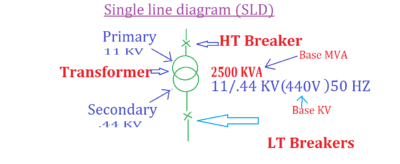# How to calculate electrical fault or Short circuit current?

## Short circuit current calculation.

Purpose

It plays a very important role to protect & also safety of commercial and industrial electrical equipment like circuit breakers,transformers …etc.

## What is short circuit current?

When two or more conductors are different phases come in contact with each other in a line, transformer or any other power element, then the part of the impedance is shunted out of the circuit due to which a large current flow in the un-faulted phases, such current is called the short circuit current.

It reduces the effect of impedance in the circuit while the current in the circuit rises.

• The flow of large current will overheat the equipment.
• The flow of short circuit current in the current carrying parts produces a force of electrodynamics interaction which may destroy or damage the equipment.

Fault MVA    = (Base MVA / % Z  ) × 100

MVA     = KVA / 1000

Where,

Base MVA= Transformer Rating

%Z = Impedance + 10 % tolerance of z

Isc    =Fault MVA / (√3 × Base KVA )

Where,

I SC  = Value of fault current or short circuit current in KA

Base KVA= Transformer is secondary output voltage (like 6.6kv/440 v)Single lie Diagram

So let’s take Quick Observation

Calculate Fault current at each stage of following Electrical System SLD having details of.

• Transformer Rating is 2.5 MVA.
• Transformer Impedance is 7.25 %.

Solution:

Use the above  formula to convert MVA to KVA

2500/1000= 2.5 MVA

As per above formula

Fault MVA      =2.5 / (7.25 % + 10% tolerance)  ×  100

= 2.5 / (7.25 +0.725) × 100

=31.32 MVA

Now calculate Short circuit current

Isc    =     31.32 / (√3 × 0.44)    = 41.09 KA

Short circuit current  is  41.09 KA.

How to calculate Voltage drop ?

How to calculate  transformer sizing ?